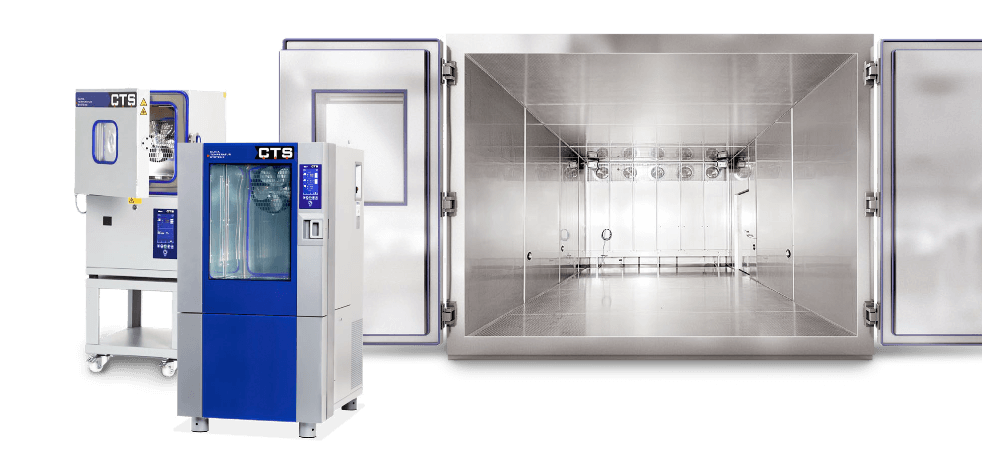General:

The value to be inputted in the commands described below has the unit of measurement K/min. The system will run with this rate of change using the set target value. In order to carry out a ramp using a target value, input the change speed first and then input the desired final value as target value. When the final value is reached, the system will continue to run with this target value until a new value is inputted.

With the ITC controller, the actual target value in the context of the ramp will always be outputted when the command to read the analogue values is given. The command 'E' (see below) is to be used to query the set target value of the ramp.

Attention:
The gradient that is set will remain in effect in the controls until a new value is set. If a run is to be carried out with the maximum target value stepping range then the gradient is to be set to a maximum value of 999.9 K/min.
Note:

The value of the gradient can also be inputted in a different format. If, for example, more precise values are necessary for the gradient then values can be inputted with two decimal places.

Example:
00.05 -> 0.05 K/min
23.45 -> 23.45 K/min